Next: Centre of mass Up: Rotational motion Previous: Is rotation a vector?

## The vector product

We saw earlier, in Sect. 3.10, that it is possible to combine two vectors multiplicatively, by means of a scalar product, to form a scalar. Recall that the scalar product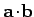of two vectors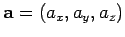and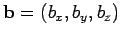is defined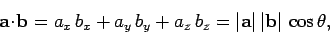(319)

whereis the angle subtended between the directions of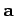and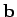.

Is it also possible to combine two vector multiplicatively to form a third (non-coplanar) vector? It turns out that this goal can be achieved via the use of the so-called vector product. By definition, the vector product,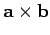, of two vectorsandis of magnitude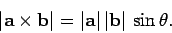(320)

The direction ofis mutually perpendicular toand, in the sense given by the right-hand grip rule when vectoris rotated onto vector(the direction of rotation being such that the angle of rotation is less than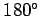). See Fig. 70. In coordinate form,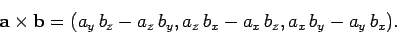(321)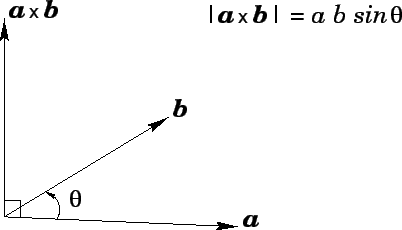There are a number of fairly obvious consequences of the above definition. Firstly, if vectoris parallel to vector, so that we can write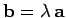, then the vector producthas zero magnitude. The easiest way of seeing this is to note that ifandare parallel then the anglesubtended between them is zero, hence the magnitude of the vector product,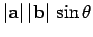, must also be zero (since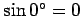). Secondly, the order of multiplication matters. Thus,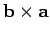is not equivalent to. In fact, as can be seen from Eq. (321),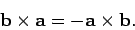(322)

In other words,has the same magnitude as, but points in diagrammatically the opposite direction.

Now that we have defined the vector product of two vectors, let us find a use for this concept. Figure 71 shows a rigid body rotating with angular velocity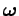. For the sake of simplicity, the axis of rotation, which runs parallel to, is assumed to pass through the originof our coordinate system. Point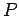, whose position vector is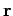, represents a general point inside the body. What is the velocity of rotationat point? Well, the magnitude of this velocity is simply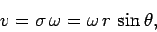(323)

where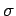is the perpendicular distance of pointfrom the axis of rotation, andis the angle subtended between the directions ofand. The direction of the velocity is into the page. Another way of saying this, is that the direction of the velocity is mutually perpendicular to the directions ofand, in the sense indicated by the right-hand grip rule whenis rotated onto(through an angle less than). It follows that we can write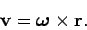(324)

Note, incidentally, that the direction of the angular velocity vectorindicates the orientation of the axis of rotation--however, nothing actually moves in this direction; in fact, all of the motion is perpendicular to the direction of.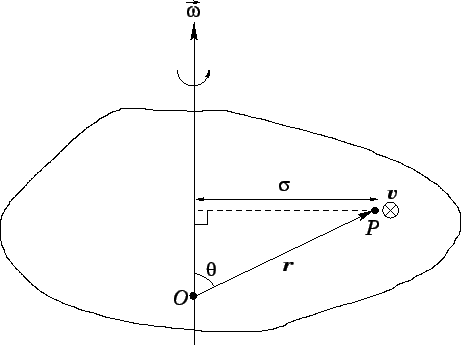Next: Centre of mass Up: Rotational motion Previous: Is rotation a vector?
Richard Fitzpatrick 2006-02-02